# Tag: puzzle

Sunday january 2nd around 18hr NeB-stats went crazy.

Referrals clarified that the post ‘What is the knot associated to a prime?’ was picked up at Reddit/math and remained nr.1 for about a day.

Now, the dust has settled, so let’s learn from the experience.A Reddit-mention is to a blog what doping is to a sporter.

You get an immediate boost in the most competitive of all blog-stats, the number of unique vistors (blue graph), but is doesn’t result in a long-term effect, and, it may even be harmful to more essential blog-stats, such as the average time visitors spend on your site (yellow graph).

For NeB the unique vistors/day fluctuate normally around 300, but peaked to 1295 and 1733 on the ‘Reddit-days’. In contrast, the avg. time on site is normally around 3 minutes, but dropped the same days to 44 and 30 seconds!

Whereas some of the Reddits spend enough time to read the post and comment on it, the vast majority zap from one link to the next. Having monitored the Reddit/math page for two weeks, I’m convinced that post only made it because it was visually pretty good. The average Reddit/math-er is a viewer more than a reader…

So, should I go for shorter, snappier, more visual posts?

Let’s compare Reddits to those coming from the three sites giving NeB most referrals : Google search, MathOverflow and Wikipedia.This is the traffic coming from Reddit/math, as always the blue graph are the unique visitors, the yellow graph their average time on site, blue-scales to the left, yellow-scales to the right.

Here’s the same graph for Google search. The unique visitors/day fluctuate around 50 and their average time on site about 2 minutes.The math-related search terms most used were this month : ‘functor of point approach’, ‘profinite integers’ and ‘bost-connes sytem’.

More rewarding to me are referrals from MathOverflow.The number of visitors depends on whether the MathO-questions made it to the front-page (for example, the 80 visits on december 15, came from the What are dessins d’enfants?-topic getting an extra comment that very day, and having two references to NeB-posts : The best rejected proposal ever and Klein’s dessins d’enfant and the buckyball), but even older MathO-topics give a few referrals a day, and these people sure take their time reading the posts (+ 5 minutes).

Other MathO-topics giving referrals this month were Most intricate and most beautiful structures in mathematics (linking to Looking for F-un), What should be learned in a first serious schemes course? (linking to Mumford’s treasure map (btw. one of the most visited NeB-posts ever)), How much of scheme theory can you visualize? (linking again to Mumford’s treasure map) and Approaches to Riemann hypothesis using methods outside number theory (linking to the Bost-Connes series).

Finally, there’s Wikipediagiving 5 to 10 referrals a day, with a pretty good time-on-site average (around 4 minutes, peaking to 12 minutes). It is rewarding to see NeB-posts referred to in as diverse Wikipedia-topics as ‘Fifteen puzzle’, ‘Field with one element’, ‘Evariste Galois’, ‘ADE classification’, ‘Monster group’, ‘Arithmetic topology’, ‘Dessin d’enfant’, ‘Groupoid’, ‘Belyi’s theorem’, ‘Modular group’, ‘Cubic surface’, ‘Esquisse d’un programme’, ‘N-puzzle’, ‘Shabat polynomial’ and ‘Mathieu group’.

What lesson should be learned from all this data? Should I go for shorter, snappier and more visual posts, or should I focus on the small group of visitors taking their time reading through a longer post, and don’t care about the appallingly high bounce rate the others cause?

To mark the end of 2009 and 6 years of blogging, two musical compositions with a mathematical touch to them. I wish you all a better 2010!

Remember from last time that we identified Olivier Messiaen as the ‘Monsieur Modulo’ playing the musical organ at the Bourbaki wedding. This was based on the fact that his “modes à transposition limitée” are really about epimorphisms between modulo rings Z/12Z→Z/3Z and Z/12Z→Z/4Z.

However, Messiaen had more serious mathematical tricks up his sleeve. In two of his compositions he did discover (or at least used) one of the smaller sporadic groups, the Mathieu group $M_{12}$ of order 95040 on which we have based a whole series of Mathieu games two and a half years ago.

Messiaen’s ‘Ile de fey 2’ composition for piano (part of Quatre études de rythme (“Four studies in rhythm”), piano (1949–50)) is based on two concurrent permutations. The first is shown below, with the underlying motive rotational permutation shown.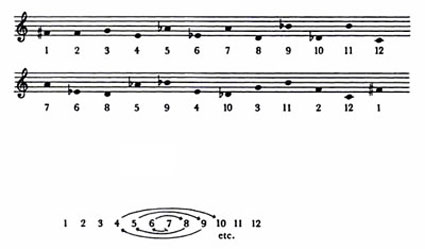This gives the permutation (1,7,10,2,6,4,5,9,11,12)(3,8). A second concurrent permutation is based on the permutation (1,6,9,2,7,3,5,4,8,10,11) and both of them generate the Mathieu group $M_{12}$. This can be seen by realizing the two permutations as the rotational permutations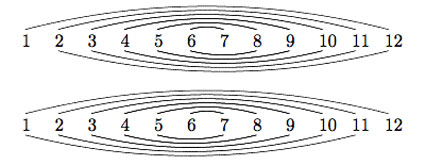and identifying them with the Mongean shuffles generating $M_{12}$. See for example, Dave Benson’s book “Music: A Mathematical Offering”, freely available online.

Clearly, Messiaen doesn’t use all of its 95040 permutations in his piece! Here’s how it sounds. The piece starts 2 minutes into the clip.

The second piece is “Les Yeux dans les Roues” (The Eyes in the Wheels), sixth piece from the “Livre d’Orgue” (1950/51).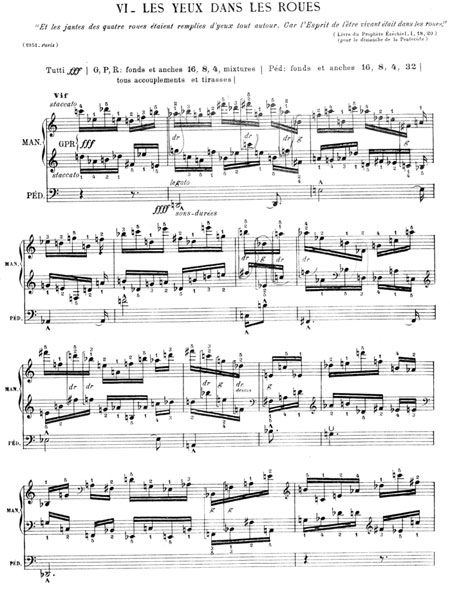According to Hauptwerk, the piece consists of a melody/theme in the pedal, accompanied by two fast-paced homorhythmic lines in the manuals. The pedal presents a sons-durées theme which is repeated six times, in different permutations. Initially it is presented in its natural form. Afterwards, it is presented alternatively picking notes from each end of the original form. Similar transformations are applied each time until the sixth, which is the retrograde of the first. The entire twelve-tone analysis (pitch only, not rhythm) of the pedal is shown below: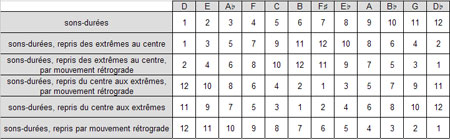That is we get the following five permutations which again generate Mathieu 12 :

• a=(2,3,5,9,8,10,6,11,4,7,12)
• b=(1,2,4,8,9,7,11,3,6,12)(5,10)=e*a
• c=(1,12,11,9,5,4,6,2,10,7)(3,8)=e*d
• d=(1,11,10,8,4,5,3,7,2,9,6)
• e=(1,12)(2,11)(3,10)(4,9)(5,8)(6,7)

Here’s the piece performed on organ :

Considering the permutations $X=d.a^{-1}$ and $Y=(a.d^2.a.d^3)^{-1}$ one obtains canonical generators of $M_{12}$, that is, generators satisfying the defining equations of this sporadic group

$X^2=Y^3=(XY)^{11}=[X,Y]^6=(XYXYXY^{-1})^6=1$

I leave you to work out the corresponding dessin d’enfant tonight after a couple of glasses of champagne! It sure has a nice form. Once again, a better 2010!

It’s great fun trying to decode some of the puns contained in Betti Bourbaki’s wedding invitation. Below a photograph, taken on May 13th 1939, of three of the practical jokers (from left to right : Ralph Boas, Frank Smithies and Andre Weil), the others were Claude Chabauty, Weil’s wife Eveline and Louis Bouckaert (from Louvain).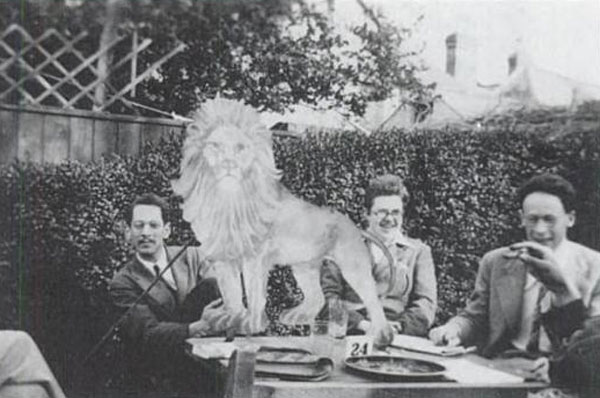Part of this picture is on the front cover of the book Lion Hunting & other Mathematical Pursuits. This book clarifies the ‘Secrétaire de l’Oevre du Sou du Lion’-phrase as well as some of the names on the card.

Inspired by the Bourbaki-hoax, a group of postdoctoral fellows visiting Princeton University in 1937-1938 (Boas, Smithies and John Tuckey) published their inventions, allegedly devised by Hector Pétard (aka ‘H(oist) W(ith) O(wn) Petard’ after the Shakespeare line “For ’tis the sport to have the engineer, hoist with his own petard…” Hamlet act III scene IV) who was writing under the pseudonym of E.S. Pondiczery. Pétard’s existence was asserted in the paper “A Contribution to the Mathematical Theory of Big-Game Hunting” Amer. Math. Monthly 45 (1938) 446-447.

Smithies recalls the spring 1939 period in Cambridge as follows : “The climax of the academic year, as far as we were concerned, came in the Easter term. André Weil, Claude Chabauty, and Louis Bouckaert (from Louvain) were all in Cambridge, and the proposal was mooted that a marriage should be arranged between Bourbaki’s daughter Betti and Hector Pétard; the marriage announcement was duly printed in the canonical French style – on it Pétard was described as the ward of Ersatz Stanislas Pondiczery – and it was circulated to the friends of both parties. A couple of weeks later the Weils, Louis Bouckaert, Max Krook (a South African astrophysicist), Ralph and myself made a river excursion to Grantchester by punt and canoe to have tea at the Red Lion; there is a photograph of Ralph and myself, with our triumphantly captured lion between us and André Weil looking benevolently on.”

From this and the date of the photograph (May 13th 1939) one can conclude that the marriage-card was drawn up around mid april 1939. As weddings tend to follow their announcement by a couple of months, this contradicts the following passage from Notice sur la Vie et l ‘oeuvre de Nicolas Bourbaki by an unidentified author :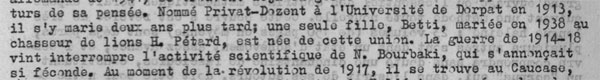“Nominated as Privat-Dozent at the University of Dorpat in 1913, he (that is, N. Bourbaki) married two years later; a single girl, Betti, married in 1938 to the Lion hunter H. Pétard, was born out of this marriage.”

But then, when was the Bourbaki-Pétard wedding scheduled? Surely, a wedding announcement should provide that information. Here’s the relevant part :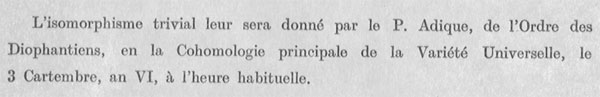“The trivial isomorphism (aka the sacrament of matrimony) will be given to them by P. Adic, of the Diophantine Order, at the Principal Cohomology of the Universal Variety, the 3 Cartember, year VI, at the usual hour.”

Here’s my guess : the first Bourbaki-meeting took place December 10th 1934. Actually, it was a ‘proto-Bourbaki-meeting’, but nevertheless founding members such as [Jean Delsarte counted 1934 as the first Bourbaki year as is clear from the ‘Remarque’ at the top of his notes of the first meeting : 34+25=59, trying to figure out when the 25-year festivity was going to be held …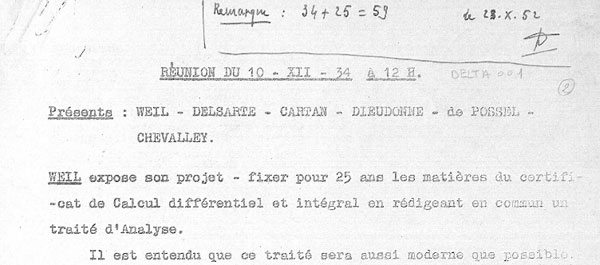Thus, if 1934 is year 1 of the Bourbaki-calender, year VI should be 1939. The notules also give a hint of ‘the usual hour’. In the 1934-1940 period, the Bourbakis met twice a month before the monday-afternoon seminar, at 12 o’clock sharp, the ‘sacred hour’, for a meeting over lunch.

Remains the ‘Cartembre’-puzzle. We know ‘Septembre (7), Novembre (9), Decembre (10)’ so if ‘Cart’ is short for ‘Quatre’ (4), Cartembre might be June. I guess the wedding was scheduled to be held on June 3rd, 1939 at 12h.

It fits with the date the announcement was drawn up and June 3rd, 1939 sure enough was a saturday, the ‘canonical’ day for a wedding. Remains the problem of the wedding place. Suggestions anyone?

Two questions from my last group-theory 101 exam:

(a) : What are the Jordan-Holder components of the Abelian group $\mathbb{Z}/20 \mathbb{Z}$?

(b) : Determine the number of order 7 elements in a simple group of order 168.

Give these to any group of working mathematicians, and, I guess all of them will solve (a), whereas the number of correct solutions to (b) will be (substantially) smaller.

Guess what? All(!) my students solved (b) correctly, whereas almost none of them had anything sensible to say about (a). A partial explanation is that they had more drill-exercises applying the Sylow-theorems than ones concerning the Jordan-Holder theorem.

A more fundamental explanation is that (b) has to do with sub-structures whereas (a) concerns quotients. Over the years I’ve tried numerous methods to convey the quotient-idea : putting things in bags, dividing a big group-table into smaller squares, additional lessons on relations, counting modulo numbers … No method appears to have an effect, lasting until the examination.

At the moment I’m seriously considering to rewrite the entire course, ditching quotients and using them only in disguise via groupoids. Before you start bombarding me with comments, I’m well aware of the problems inherent in this approach.

Before you do groupoids, students have to know some basic category theory. But that’s ok with me. Since last year it has been decided that I should sacrifice the first three weeks of the course telling students the basics of sets, maps and relations. After this, the formal definition of a category will appear more natural to them than the definition of a group, not? Besides, most puzzle-problems I use to introduce groups are actually examples of groupoids…

But then, what are the main theorems on finite groupoids? Well, I can see the groupoid cardinality result, giving you in one stroke Lagrange’s theorem as well as the orbit-counting method. From this one can then prove the remaining classical group-results such as Cauchy and the Sylows, but perhaps there are more elegant approaches?

Have you seen a first-year group-theory course starting off with groupoids? Do you know an elegant way to prove a classical group-result using groupoids?

About a year ago I did a series of posts on games associated to the Mathieu sporadic group $M_{12}$, starting with a post on Conway’s puzzle M(13), and, continuing with a discussion of mathematical blackjack. The idea at the time was to write a book for a general audience, as discussed at the start of the M(13)-post, ending with a series of new challenging mathematical games. I asked : “What kind of puzzles should we promote for mathematical thinking to have a fighting chance to survive in the near future?”

Now, Scientific American has (no doubt independently) taken up this lead. Their July 2008 issue features the article Rubik’s Cube Inspired Puzzles Demonstrate Math’s “Simple Groups” written by Igor Kriz and Paul Siegel.

By far the nicest thing about this article is that it comes with three online games based on the sporadic simple groups, the Mathieu groups $M_{12}$, $M_{24}$ and the Conway group $.0$.

the M(12) game

Scrambles to an arbitrary permutation in $M_{12}$ and need to use the two generators $INVERT=(1,12)(2,11)(3,10)(4,9)(5,8)(6,7)$ and $MERGE=(2,12,7,4,11,6,10,8,9,5,3)$ to return to starting position.Here is the help-screen :They promise the solution by july 27th, but a few-line GAP-program cracks the puzzle instantly.

the M(24) game

Similar in nature, again using two generators of $M_{24}$. GAP-solution as before.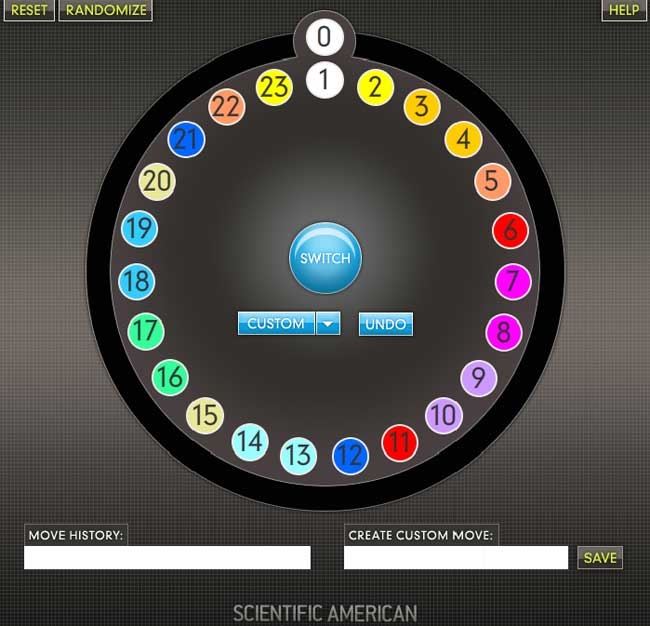This time, they offer this help-screen :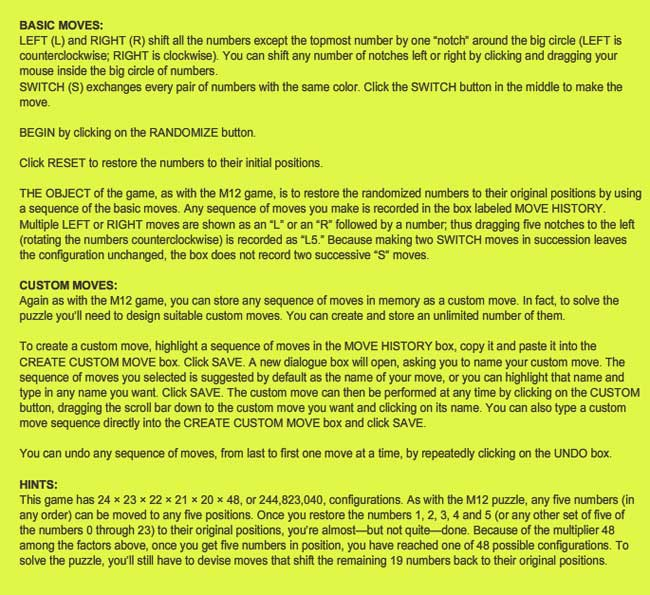the .0 game

Their most original game is based on Conway’s $.0$ (dotto) group. Unfortunately, they offer only a Windows-executable version, so I had to install Bootcamp and struggle a bit with taking screenshots on a MacBook to show you the game’s starting position :Dotto:

Dotto, our final puzzle, represents the Conway group Co0, published in 1968 by mathematician John H. Conway of Princeton University. Co0 contains the sporadic simple group Co1 and has exactly twice as many members as Co1. Conway is too modest to name Co0 after himself, so he denotes the group “.0” (hence the pronunciation “dotto”).

In Dotto, there are four moves. This puzzle includes the M24 puzzle. Look at the yellow/blue row in the bottom. This is, in fact, M24, but the numbers are arranged in a row instead of a circle. The R move is the “circle rotation to the right”: the column above the number 0 stays put, but the column above the number 1 moves to the column over the number 2 etc. up to the column over the number 23, which moves to the column over the number 1. You may also click on a column number and then on another column number in the bottom row, and the “circle rotation” moving the first column to the second occurs. The M move is the switch, in each group of 4 columns separated by vertical lines (called tetrads) the “yellow” columns switch and the “blue” columns switch. The sign change move (S) changes signs of the first 8 columns (first two tetrads). The tetrad move (T) is the most complicated: Subtract in each row from each tetrad 1/2 times the sum of the numbers in that tetrad. Then in addition to that, reverse the signs of the columns in the first tetrad.

Strategy hints: Notice that the sum of squares of the numbers in each row doesn’t change. (This sum of squares is 64 in the first row, 32 in every other row.) If you manage to get an “8”in the first row, you have almost reduced the game to M24 except those signs. To have the original position, signs of all numbers on the diagonal must be +. Hint on signs: if the only thing wrong are signs on the diagonal, and only 8 signs are wrong, those 8 columns can be moved to the first 8 columns by using only the M24 moves (M,R).

The buckyball is without doubt the hottest mahematical object at the moment (at least in Europe). Recall that the buckyball (middle) is a mixed form of two Platonic solids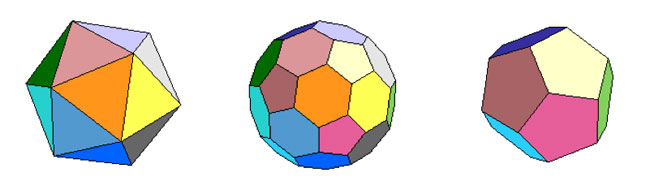the Icosahedron on the left and the Dodecahedron on the right.

For those of you who don’t know anything about football, it is that other ball-game, best described via a quote from the English player Gary Lineker

“Football is a game for 22 people that run around, play the ball, and one referee who makes a slew of mistakes, and in the end Germany always wins.”

We still have a few days left hoping for a better ending… Let’s do some bucky-maths : what is the rotation symmetry group of the buckyball?

For starters, dodeca- and icosahedron are dual solids, meaning that if you take the center of every face of a dodecahedron and connect these points by edges when the corresponding faces share an edge, you’ll end up with the icosahedron (and conversely). Therefore, both solids (as well as their mixture, the buckyball) will have the same group of rotational symmetries. Can we at least determine the number of these symmetries?

Take the dodecahedron and fix a face. It is easy to find a rotation taking this face to anyone of its five adjacent faces. In group-slang : the rotation automorphism group acts transitively on the 12 faces of the dodecohedron. Now, how many of them fix a given face? These can only be rotations with axis through the center of the face and there are exactly 5 of them preserving the pentagonal face. So, in all we have $12 \times 5 = 60$ rotations preserving any of the three solids above. By composing two of its elements, we get another rotational symmetry, so they form a group and we would like to determine what that group is.

There is one group that springs to mind $A_5$, the subgroup of all even permutations on 5 elements. In general, the alternating group has half as many elements as the full permutation group $S_n$, that is $\frac{1}{2} n!$ (for multiplying with the involution (1,2) gives a bijection between even and odd permutations). So, for $A_5$ we get 60 elements and we can list them :

• the trivial permutation$~()$, being the identity.
• permutations of order two with cycle-decompostion $~(i_1,i_2)(i_3,i_4)$, and there are exactly 15 of them around when all numbers are between 1 and 5.
• permutations of order three with cycle-form $~(i_1,i_2,i_3)$ of which there are exactly 20.
• permutations of order 5 which have to form one full cycle $~(i_1,i_2,i_3,i_4,i_5)$. There are 24 of those.

Can we at least view these sets of elements as rotations of the buckyball? Well, a dodecahedron has 12 pentagobal faces. So there are 4 nontrivial rotations of order 5 for every 2 opposite faces and hence the dodecaheder (and therefore also the buckyball) has indeed 6×4=24 order 5 rotational symmetries.

The icosahedron has twenty triangles as faces, so any of the 10 pairs of opposite faces is responsible for two non-trivial rotations of order three, giving us 10×2=20 order 3 rotational symmetries of the buckyball.

The order two elements are slightly harder to see. The icosahedron has 30 edges and there is a plane going through each of the 15 pairs of opposite edges splitting the icosahedron in two. Hence rotating to interchange these two edges gives one rotational symmetry of order 2 for each of the 15 pairs.

And as 24+20+15+1(identity) = 60 we have found all the rotational symmetries and we see that they pair up nicely with the elements of $A_5$. But do they form isomorphic groups? In other words, can the buckyball see the 5 in the group $A_5$.

In a previous post I’ve shown that one way to see this 5 is as the number of inscribed cubes in the dodecahedron. But, there is another way to see the five based on the order 2 elements described before.

If you look at pairs of opposite edges of the icosahedron you will find that they really come in triples such that the planes determined by each pair are mutually orthogonal (it is best to feel this on ac actual icosahedron). Hence there are 15/3 = 5 such triples of mutually orthogonal symmetry planes of the icosahedron and of course any rotation permutes these triples. It takes a bit of more work to really check that this action is indeed the natural permutation action of $A_5$ on 5 elements.

Having convinced ourselves that the group of rotations of the buckyball is indeed the alternating group $A_5$, we can reverse the problem : can the alternating group $A_5$ see the buckyball???

Well, for starters, it can ‘see’ the icosahedron in a truly amazing way. Look at the conjugacy classes of $A_5$. We all know that in the full symmetric group $S_n$ elements belong to the same conjugacy class if and only if they have the same cycle decomposition and this is proved using the fact that the conjugation f a cycle $~(i_1,i_2,\ldots,i_k)$ under a permutation $\sigma \in S_n$ is equal to the cycle $~(\sigma(i_1),\sigma(i_2),\ldots,\sigma(i_k))$ (and this gives us also the candidate needed to conjugate two partitions into each other).

Using this trick it is easy to see that all the 15 order 2 elements of $A_5$ form one conjugacy class, as do the 20 order 3 elements. However, the 24 order 5 elements split up in two conjugacy classes of 12 elements as the permutation needed to conjugate $~(1,2,3,4,5)$ to $~(1,2,3,5,4)$ is $~(4,5)$ but this is not an element of $A_5$.

Okay, now take one of these two conjugacy classes of order 5 elements, say that of $~(1,2,3,4,5)$. It consists of 12 elements, 12 being also the number of vertices of the icosahedron. So, is there a way to identify the elements in the conjugacy class to the vertices in such a way that we can describe the edges also in terms of group-computations in $A_5$?

Surprisingly, this is indeed the case as is demonstrated in a marvelous paper by Kostant “The graph of the truncated icosahedron and the last letter of Galois”.

Two elements $a,b$ in the conjugacy class C share an edge if and only if their product $a.b \in A_5$ still belongs to the conjugacy class C!

So, for example $~(1,2,3,4,5).(2,1,4,3,5) = (2,5,4)$ so there is no edge between these elements, but on the other hand $~(1,2,3,4,5).(5,3,4,1,2)=(1,5,2,4,3)$ so there is an edge between these! It is no coincidence that $~(5,3,4,1,2)=(2,1,4,3,5)^{-1}$ as inverse elements correspond in the bijection to opposite vertices and for any pair of non-opposite vertices of an icosahedron it is true that either they are neighbors or any one of them is the neighbor of the opposite vertex of the other element.

If we take $u=(1,2,3,4,5)$ and $v=(5,3,4,1,2)$ (or any two elements of the conjugacy class such that u.v is again in the conjugacy class), then one can describe all the vertices of the icosahedron group-theoretically as follows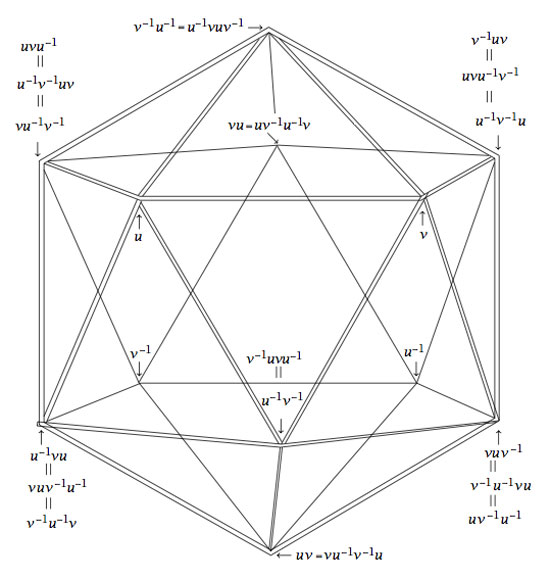Isn’t that nice? Well yes, you may say, but that is just the icosahedron. Can the group $A_5$ also see the buckyball?

Well, let’s try a similar strategy : the buckyball has 60 vertices, exactly as many as there are elements in the group $A_5$. Is there a way to connect certain elements in a group according to fixed rules? Yes, there is such a way and it is called the Cayley Graph of a group. It goes like this : take a set of generators ${ g_1,\ldots,g_k }$ of a group G, then connect two group element $a,b \in G$ with an edge if and only if $a = g_i.b$ or $b = g_i.a$ for some of the generators.

Back to the alternating group $A_5$. There are several sets of generators, one of them being the elements ${ (1,2,3,4,5),(2,3)(4,5) }$. In the paper mentioned before, Kostant gives an impressive group-theoretic proof of the fact that the Cayley-graph of $A_5$ with respect to these two generators is indeed the buckyball!

Let us allow to be lazy for once and let SAGE do the hard work for us, and let us just watch the outcome. Here’s how that’s done

A=PermutationGroup([‘(1,2,3,4,5)’,'(2,3)(4,5)’])
B=A.cayley_graph()
B.show3d()

The outcone is a nice 3-dimensional picture of the buckyball. Below you can see a still, and, if you click on it you will get a 3-dimensional model of it (first click the ‘here’ link in the new window and then you’d better control-click and set the zoom to 200% before you rotate it)Hence, viewing this Cayley graph from different points we have convinced ourselves that it is indeed the buckyball. In fact, most (truncated) Platonic solids appear as Cayley graphs of groups with respect to specific sets of generators. For later use here is a (partial) survey (taken from Jaap’s puzzle page)Tetrahedron : $C_2 \times C_2,[(12)(34),(13)(24),(14)(23)]$
Cube : $D_4,[(1234),(13)]$
Octahedron : $S_3,[(123),(12),(23)]$
Dodecahedron : IMPOSSIBLE
Icosahedron : $A_4,[(123),(234),(13)(24)]$Truncated tetrahedron : $A_4,[(123),(12)(34)]$
Cuboctahedron : $A_4,[(123),(234)]$
Truncated cube : $S_4,[(123),(34)]$
Truncated octahedron : $S_4,[(1234),(12)]$
Rhombicubotahedron : $S_4,[(1234),(123)]$
Rhombitruncated cuboctahedron : IMPOSSIBLE
Snub cuboctahedron : $S_4,[(1234),(123),(34)]$Icosidodecahedron : IMPOSSIBLE
Truncated dodecahedron : $A_5,[(124),(23)(45)]$
Truncated icosahedron : $A_5,[(12345),(23)(45)]$
Rhombicosidodecahedron : $A_5,[(12345),(124)]$
Rhombitruncated icosidodecahedron : IMPOSSIBLE
Snub Icosidodecahedron : $A_5,[(12345),(124),(23)(45)]$

Again, all these statements can be easily verified using SAGE via the method described before. Next time we will go further into the Kostant’s group-theoretic proof that the buckyball is the Cayley graph of $A_5$ with respect to (2,5)-generators as this calculation will be crucial in the description of the buckyball curve, the genus 70 Riemann surface discovered by David Singerman and
Pablo Martin which completes the trinity corresponding to the Galois trinityEver tried a chess problem like : White to move, mate in two! Of course you have, and these are pretty easy to solve : you only have to work through the finite list of white first moves and decide whether or not black has a move left preventing mate on the next white move. This is even a (non-optimal) fool-proof algorithm to find the solution to this kind of chess problems. Right?

Wrong! There exist concrete positions, provable mate in two in which it is NOT possible to determine the winning first move for white! So, what’s wrong with the argument above? We did assume that, given the position, it is possible to determine all legal moves for the two players. So?

Well, some moves are legal only depending on the history of the game. For example, you are only allowed to do a castling if your king nor your rook made a prior move. Further, you can only make an en-passant-capture on the next move.

But surely all this is just theoretical? No-one ever constructed a provable 2-mate with impossible winning move. Wrong again. The logician Raymond Smullyan did precisely that in his retro-chess puzzle book Chess mysteries of Sherlock Holmes. Here’s the position :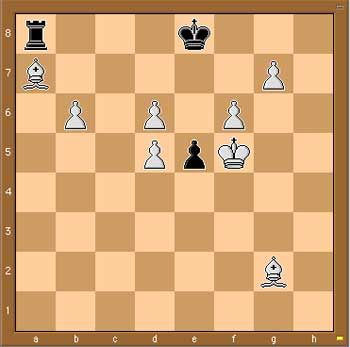Presumably every chess player goes for the mate : 1. Kf5-e6 2. g7-g8D But what if black counters your first move with a castling 1. … 0-0-0 ? Surely he isnt allowed to do this. Why not, is there any clue in the position to prove that either the king or the rook must have moved before?

Well, what was black previous move then? It cannot be the pawn move e6-e5 as before that move the white King would be in check, so what was it? Just one possibility left : it must have been e7-e5.

This offers then another winning strategy for white, as white can capture en-passant. 1. d5xe6 e.p. and then if black castles 1. … 0.0.0, 2. b6-b7 or is black does any other move : 2. g7-g8.

Hence, whatever the games’ history, white has a mate in two! However, looking ONLY at the given position, it is impossible for him to judge whether Kf5-e6 will do the trick!

Anyone seen similar constructions?

UPDATE

According to the wikipedia page on Retrograde chess analysis, the Smullyan-idea is an adaptation of a much older problem due to W. Langstaff in the Chess Amateur of 1922. Here’s the situation (the solution is the same as above)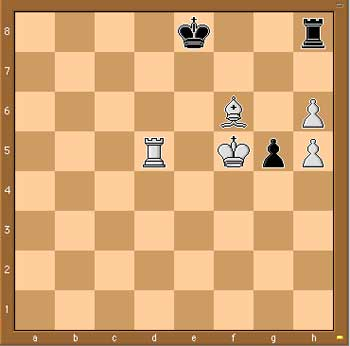MUBs (for Mutually Unbiased Bases) are quite popular at the moment. Kea is running a mini-series Mutual Unbias as is Carl Brannen. Further, the Perimeter Institute has a good website for its seminars where they offer streaming video (I like their MacromediaFlash format giving video and slides/blackboard shots simultaneously, in distinct windows) including a talk on MUBs (as well as an old talk by Wootters).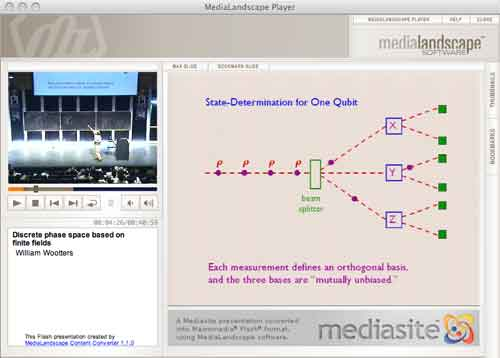So what are MUBs to mathematicians? Recall that a d-state quantum system is just the vectorspace $\mathbb{C}^d$ equipped with the usual Hermitian inproduct $\vec{v}.\vec{w} = \sum \overline{v_i} w_i$. An observable $E$ is a choice of orthonormal basis ${ \vec{e_i} }$ consisting of eigenvectors of the self-adjoint matrix $E$. $E$ together with another observable $F$ (with orthonormal basis ${ \vec{f_j} }$) are said to be mutally unbiased if the norms of all inproducts $\vec{f_j}.\vec{e_i}$ are equal to $1/\sqrt{d}$. This definition extends to a collection of pairwise mutually unbiased observables. In a d-state quantum system there can be at most d+1 mutually unbiased bases and such a collection of observables is then called a MUB of the system. Using properties of finite fields one has shown that MUBs exists whenever d is a prime-power. On the other hand, existence of a MUB for d=6 still seems to be open…

The King’s Problem (( actually a misnomer, it’s more the poor physicists’ problem… )) is the following : A physicist is trapped on an island ruled by a mean
king who promises to set her free if she can give him the answer to the following puzzle. The
physicist is asked to prepare a d−state quantum system in any state of her choosing and give it
to the king, who measures one of several mutually unbiased observables on it. Following this, the physicist is allowed to make a control measurement
on the system, as well as any other systems it may have been coupled to in the preparation
phase. The king then reveals which observable he measured and the physicist is required
to predict correctly all the eigenvalues he found.

The Solution to the King’s problem in prime power dimension by P. K. Aravind, say for $d=p^k$, consists in taking a system of k object qupits (when $p=2l+1$ one qupit is a spin l particle) which she will give to the King together with k ancilla qupits that she retains in her possession. These 2k qupits are diligently entangled and prepared is a well chosen state. The final step in finding a suitable state is the solution to a pure combinatorial problem :

She must use the numbers 1 to d to form $d^2$ ordered sets of d+1 numbers each, with repetitions of numbers within a set allowed, such that any two sets have exactly one identical number in the same place in both. Here’s an example of 16 such strings for d=4 :

11432, 12341, 13214, 14123, 21324, 22413, 23142, 24231, 31243, 32134, 33421, 34312, 41111, 42222, 43333, 44444

Here again, finite fields are used in the solution. When $d=p^k$, identify the elements of $\mathbb{F}_{p^k}$ with the numbers from 1 to d in some fixed way. Then, the $d^2$ of number-strings are found as follows : let $k_0,k_1 \in \mathbb{F}_{p^k}$ and take as the first 2 numbers the ones corresponding to these field-elements. The remaning d-2 numbers in the string are those corresponding to the field element $k_m$ (with $2 \leq m \leq d$) determined from $k_0,k_1$ by the equation

$k_m = l_{m} * k_0+k_1$

where $l_i$ is the field-element corresponding to the integer i ($l_1$ corresponds to the zero element). It is easy to see that these $d^2$ strings satisfy the conditions of the combinatorial problem. Indeed, any two of its digits determine $k_0,k_1$ (and hence the whole string) as it follows from
$k_m = l_m k_0 + k_1$ and $k_r = l_r k_0 + k_1$ that $k_0 = \frac{k_m-k_r}{l_m-l_r}$.

In the special case when d=3 (that is, one spin 1 particle is given to the King), we recover the tetracode : the nine codewords

0000, 0+++, 0—, +0+-, ++-0, +-0+, -0-+, -+0-, –+0

encode the strings (with +=1,-=2,0=3)

3333, 3111, 3222, 1312, 1123, 1231, 2321, 2132, 2213

The Archimedes codex is a good read, especially when you are (like me) a failed archeologist. The palimpsest (Greek for ‘scraped again’) is the worlds first Kyoto-approved ‘sustainable writing’. Isn’t it great to realize that one of the few surviving texts by Archimedes only made it because some monks recycled an old medieval parchment by scraping off most of the text, cutting the pages in half, rebinding them and writing a song-book on them…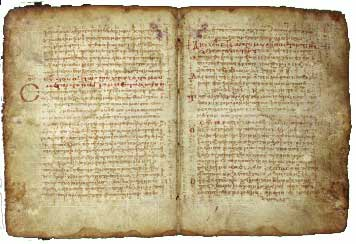The Archimedes-text is barely visible as vertical lines running through the song-lyrics. There is a great website telling the story in all its detail.

Contrary to what the books claims I don’t think we will have to rewrite maths history. Didn’t we already know that the Greek were able to compute areas and volumes by approximating them with polygons resp. polytopes? Of course one might view this as a precursor to integral calculus… And then the claim that Archimedes invented ordinal calculus. Sure the Greek knew that there were ‘as many’ even integers than integers… No, for me the major surprise was their theory about the genesis of mathematical notation.

The Greek were pure ASCII mathematicians : they wrote their proofs out in full text. Now, here’s an interesting theory how symbols got into maths… pure laziness of the medieval monks transcribing the old works! Copying a text was a dull undertaking so instead of repeating ‘has the same ratio as’ for the 1001th time, these monks introduced abbreviations like $\Sigma$ instead… and from then on things got slightly out of hand.

Another great chapter is on the stomachion, perhaps the oldest mathematical puzzle. Just a few pages made in into the palimpsest so we do not really know what (if anything) Archimedes had to say about it, but the conjecture is that he was after the number of different ways one could make a square with the following 14 pieces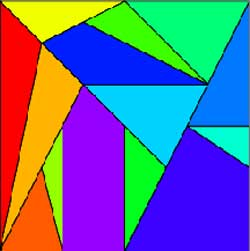People used computers to show that the total number is $17152=2^8 \times 67$. The 2-power is hardly surprising in view of symmetries of the square (giving $8$) and the fact that one can flip one of the two vertical or diagonal parts in the alternative description of the squarebut I sure would like to know where the factor 67 is coming from… The MAA and UCSD have some good pages related to the stomachion puzzle. Finally, the book also views the problema bovinum as an authentic Archimedes, so maybe I should stick to my promise to blog about it, after all…

Below an up-till-now hidden post, written november last year, trying to explain the long blog-silence at neverendingbooks during october-november 2007…

A couple of months ago a publisher approached me, out of the blue, to consider writing a book about mathematics for the general audience (in Dutch (?!)). Okay, I brought this on myself hinting at the possibility in this post

Recently, I’ve been playing with the idea of writing a book for the general public. Its title is still unclear to me (though an idea might be “The disposable science”, better suggestions are of course wellcome) but I’ve fixed the subtitle as “Mathematics’ puzzling fall from grace”. The book’s concept is simple : I would consider the mathematical puzzles creating an hype over the last three centuries : the 14-15 puzzle for the 19th century, Rubik’s cube for the 20th century and, of course, Sudoku for the present century.

For each puzzle, I would describe its origin, the mathematics involved and how it can be used to solve the puzzle and, finally, what the differing quality of these puzzles tells us about mathematics’ changing standing in society over the period. Needless to say, the subtitle already gives away my point of view. The final part of the book would then be more optimistic. What kind of puzzles should we promote for mathematical thinking to have a fighting chance to survive in the near future?

While I still like the idea and am considering the proposal, chances are low this book ever materializes : the blog-title says it all…

Then, about a month ago I got some incoming links from a variety of Flemish blogs. From their posts I learned that the leading Science-magazine for the low countries, Natuur, Wetenschap & Techniek (Nature, Science & Technology), featured an article on Flemish science-blogs and that this blog might be among the ones covered. It sure would explain the publisher’s sudden interest. Of course, by that time the relevant volume of NW&T was out of circulation so I had to order a backcopy to find out what was going on. Here’s the relevant section, written by their editor Erick Vermeulen (as well as an attempt to translate it)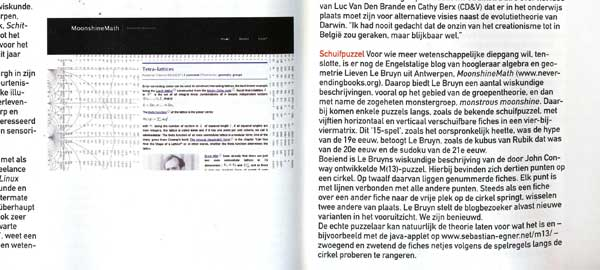Sliding puzzle For those who want more scientific depth (( their interpretation, not mine )), there is the English blog by Antwerp professor algebra & geometry Lieven Le Bruyn, MoonshineMath (( indicates when the article was written… )). Le Bruyn offers a number of mathematical descriptions, most of them relating to group theory and in particular the so called monster-group and monstrous moonshine. He mentions some puzzles in passing such as the well known sliding puzzle with 15 pieces sliding horizontally and vertically in a 4 by 4 matrix. Le Bruyn argues that this ’15-puzzle (( The 15-puzzle groupoid ))’ was the hype of the 19th century as was the Rubik cube for the 20th and is Sudoku for the 21st century.
Interesting is Le Bruyn’s mathematical description of the M(13)-puzzle (( Conway’s M(13)-puzzle )) developed by John Conway. It has 13 points on a circle, twelve of them carrying a numbered counter. Every point is connected via lines to all others (( a slight simplification )). Whenever a counter jumps to the empty spot, two others exchange places. Le Bruyn promises the blog-visitor new variants to come (( did I? )). We are curious.
Of course, the genuine puzzler can leave all this theory for what it is, use the Java-applet (( Egner’s M(13)-applet )) and painfully try to move the counters around the circle according to the rules of the game.

Some people crave for this kind of media-attention. On me it merely has a blocking-effect. Still, as the end of my first-semester courses comes within sight, I might try to shake it off…